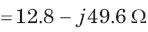Courses

# Sinusoidal Steady State Analysis - MCQ Test

## 20 Questions MCQ Test GATE ECE (Electronics) 2022 Mock Test Series | Sinusoidal Steady State Analysis - MCQ Test

Description
This mock test of Sinusoidal Steady State Analysis - MCQ Test for Railways helps you for every Railways entrance exam. This contains 20 Multiple Choice Questions for Railways Sinusoidal Steady State Analysis - MCQ Test (mcq) to study with solutions a complete question bank. The solved questions answers in this Sinusoidal Steady State Analysis - MCQ Test quiz give you a good mix of easy questions and tough questions. Railways students definitely take this Sinusoidal Steady State Analysis - MCQ Test exercise for a better result in the exam. You can find other Sinusoidal Steady State Analysis - MCQ Test extra questions, long questions & short questions for Railways on EduRev as well by searching above.
QUESTION: 1

### In a two element series circuit, the applied voltage and the resulting current are v(t) = 60 + 66sin (10 t) V, i(t) = 2.3sin (103t + 68.3o) A.The nature of the elements would be

Solution:

R-Ccauses a positive phase shift in voltage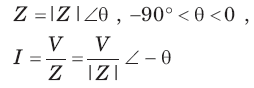QUESTION: 2

### vo (t) = ?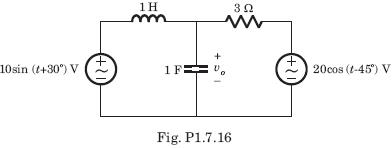Solution:

10 sin (t + 30°) = 10 cos (t - 60°)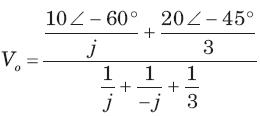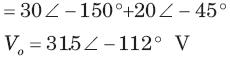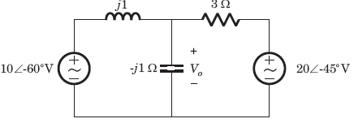QUESTION: 3

### Vo = ?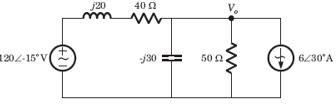Solution:
QUESTION: 4

The circuit is as shown in fig.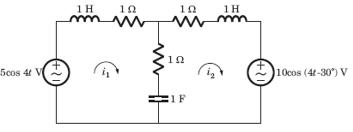i1( t) = ?

Solution: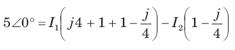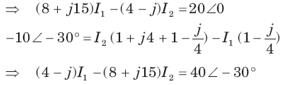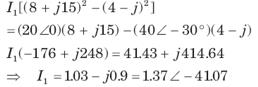QUESTION: 5

The circuit is as shown in fig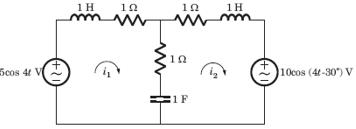i2(t) = ?

Solution: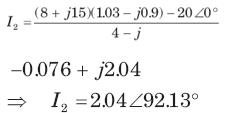QUESTION: 6

Ix = ?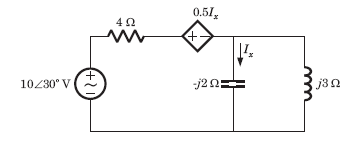Solution: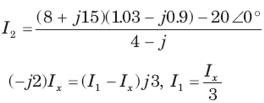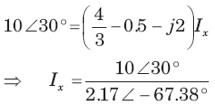QUESTION: 7

Vx = ?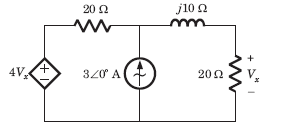Solution:

Let Vo be the voltage across current source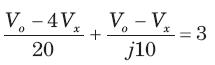Vo(20 + j10) - (20 + j40) Vx = j600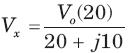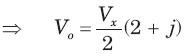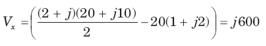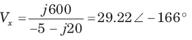QUESTION: 8

Determine the complex power for hte given values in question.

P = 269 W, Q = 150 VAR (capacitive)

Solution:

S = P-jQ = 269-j150 VA

QUESTION: 9

Determine the complex power for hte given valuesin question.

Q = 2000 VAR, pf =09. (leading)

Solution:

pf = cos θ = 0.9 ⇒ θ = 25.84°
Q = S sin θ ⇒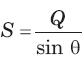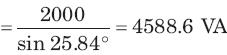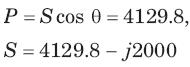QUESTION: 10

Determine the complex power for hte given values in question.

S = 60 VA, Q = 45 VAR (inductive)

Solution:

Q = S sin θ ⇒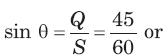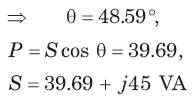QUESTION: 11

Determine the complex power for hte given values in question.

Vrms = 220 V, P = 1 kW, |Z| = 40Ω (inductive)

Solution: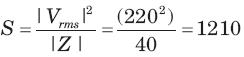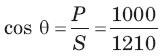= 0.8264 or θ = 34.26°,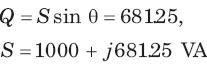QUESTION: 12

Determine the complex power for hte given values in question

Vrms = 21∠20°V, Vrms = 21∠20°V, Irms = 8.5∠-50°A

Solution:

S = Vrms I*rms = (21∠20°)(8.5∠50°)

= 61+j167.7VA

QUESTION: 13

Determine the complex power for hte given values in question.

Vrms = 120∠30°V, Z = 40 + j80Ω

Solution: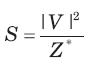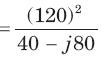= 72 + j144 VA

QUESTION: 14

In a two element series circuit, the applied voltage and the resulting current are v(t) = 60 + 66 sin (1000t) V, i(t) = 2.3sin (1000t + 68.3) 3 A. The nature of the elements would be

Solution:
QUESTION: 15

A relay coil is connected to a 210 V, 50 Hz supply. If it has resistance of 30Ω and an inductance of 0.5 H, the apparent power is

Solution:

Z= 30 + j(0.5)(2π)(50) = 30 + j157,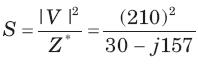Apparent power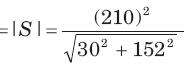= 275.6 VA

QUESTION: 16

In the circuit shown in fig. power factor is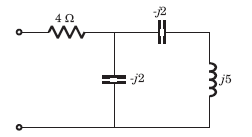Solution: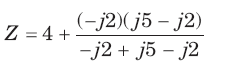= 4 - j6 = 7.21∠ - 56.31°, pf = cos 56.31° = 0.555 leading

QUESTION: 17

The power factor seen by the voltage source is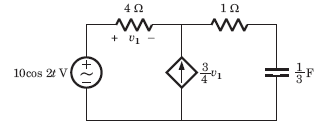Solution: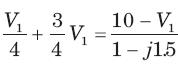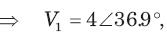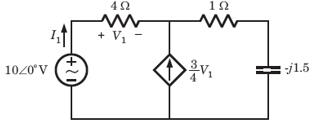I1 = 1∠36.9°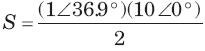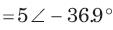pf = cos 36.9° = 0.8 leading

QUESTION: 18

The average power supplied by the dependent source is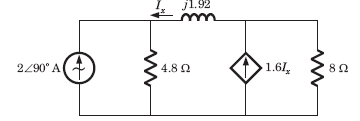Solution:

(2∠ - 90°)4.8 = -Ix (4.8 + j1.92) + 0.6Ix(8)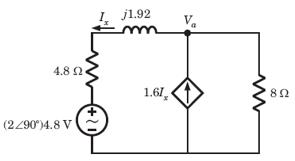Ix = 5∠0°,   Va = 0.6 x 5 x 8 = 24∠0°,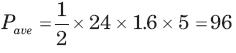QUESTION: 19

In the circuit of fig. the maximum power absorbed by ZL is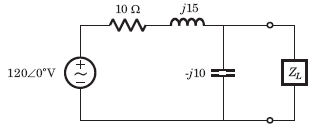Solution: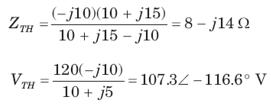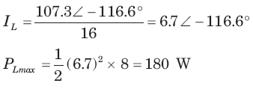QUESTION: 20

The value of the load impedance, that would absorbs the maximum average power is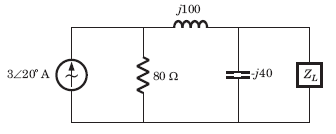Solution: